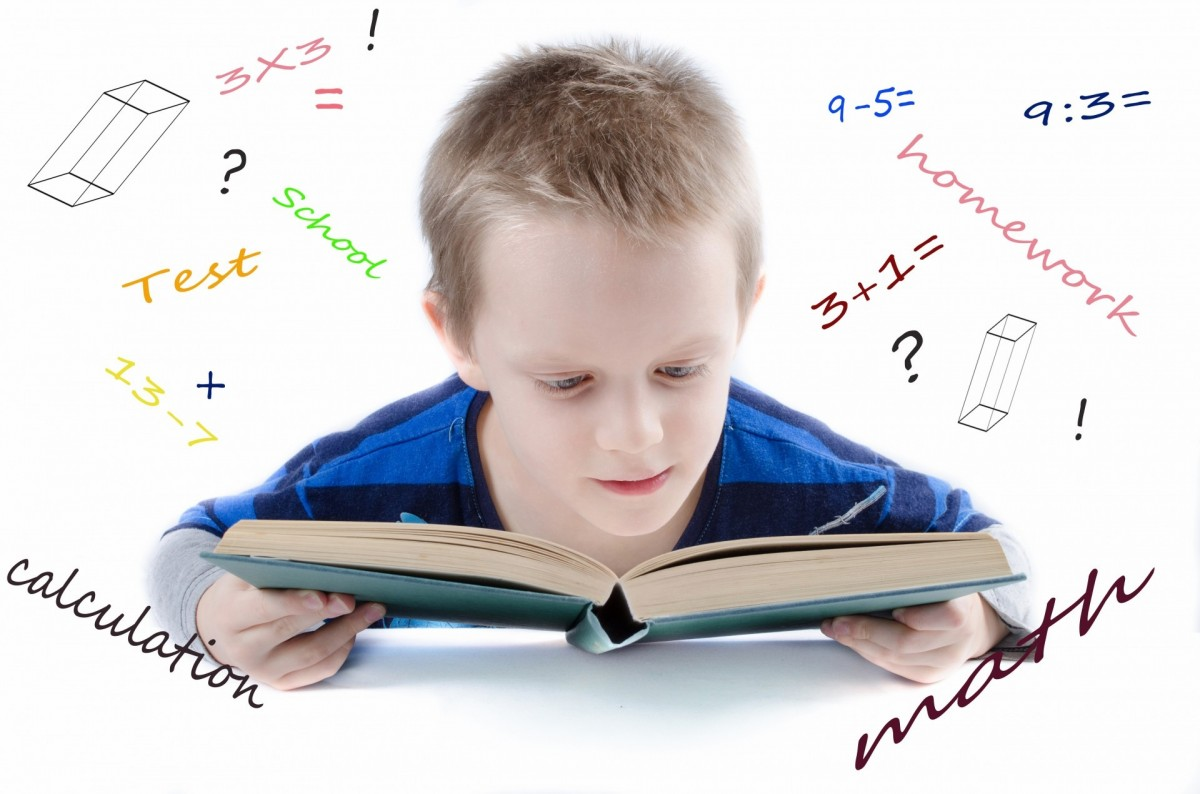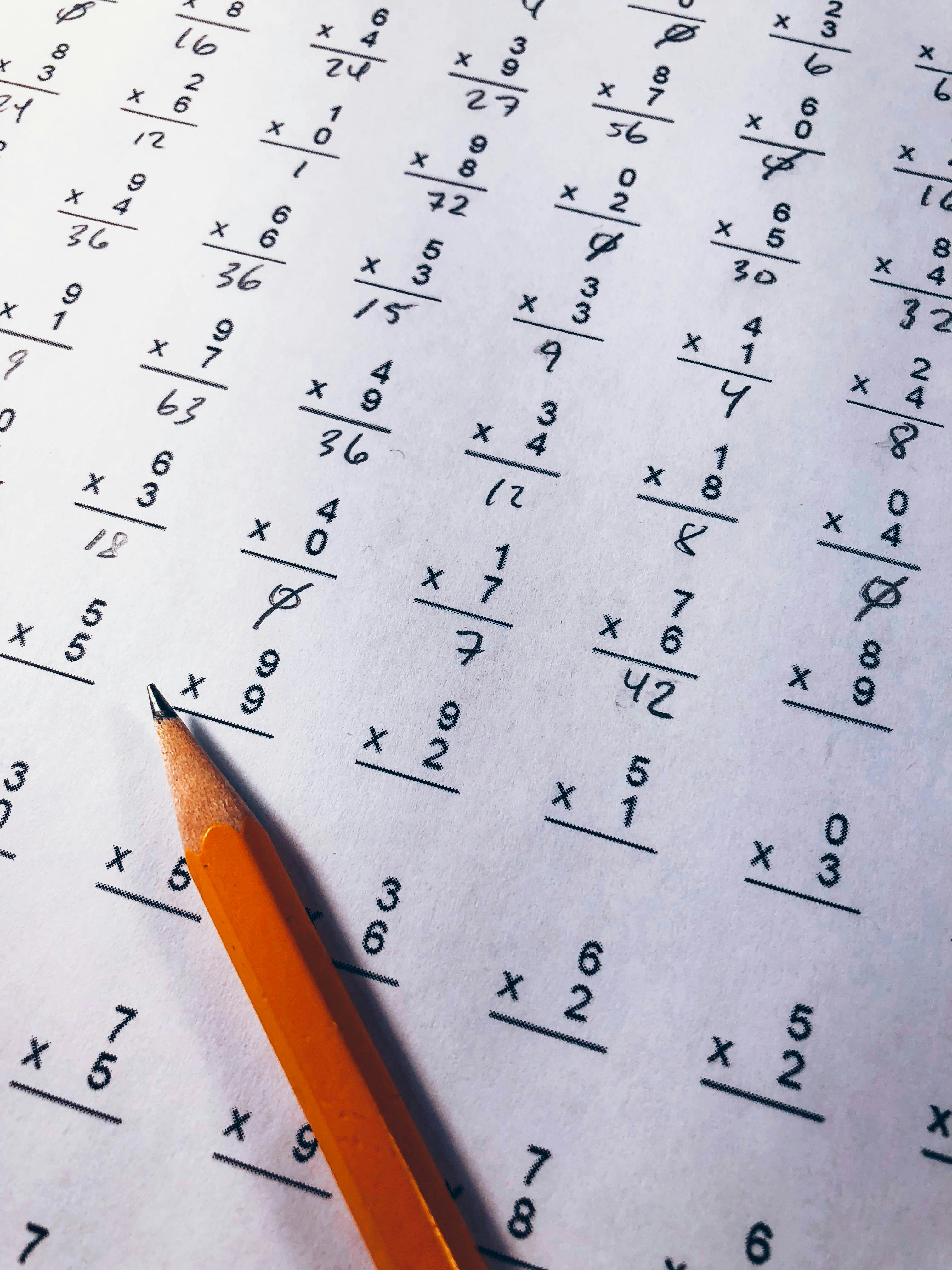# Everything you should know about Dyscalculia or Number Dyslexia.

## What is Dyscalculia (or Number Dyslexia)?

Dyscalculia is a term used for a wide range of life-long learning disabilities involving maths, mostly numbers and arithmetic skills. Other names of Dyscalculia are Number Dyslexia, Numerical Dyslexia, and Math Dyslexia. It is generally seen as the mathematical equivalent to dyslexia.

Dyscalculia is often confused with math anxiety, which has a different pathology, but shows similar signs.

Difficulty in understanding numbers, manipulating them, performing mathematical calculations and learning facts in mathematics are some of the common known traits of this disorder.

It is also marked by visual difficulties such as  processing and distinguishing visuals, pattern recognition, working-memory for numbers and formulas, retrieval of learned facts and procedures, directional confusion, processing speed and time. Perhaps, there is no single form of math disability, and difficulties vary from person to person.

According to the British Dyslexia Association, Dyscalculia is likely to occur in 3% – 6% of the population. Dyslexia and Dyscalculia relation is independent in nature. Research suggests that 40 to 50 percent of dyslexics show no signs of this number dyslexia. They perform at least as well in maths as other children, with about 10 percent achieving at a higher level. The remaining 50 to 60 percent do have difficulties with maths.

## Types of Dyscalculia

Dyscalculia usually presents itself in 5 main types.

• Verbal dyscalculia:This type of dyscalculia is characterized by a difficulty naming and understanding the mathematical concepts presented verbally. Children with this type of dyscalculia are able to read or write numbers, but have problem  recognizing them when presented verbally.

• Practognostic dyscalculia: Children with Practognostic dyscalculia have a hard time manipulating mathematics equations and  comparing real world measurements.

• Lexical dyscalculia: The part of dyscalculia referring to problems in reading mathematical symbols, including operation signs (+, – ) , numerals and mathematical expressions or equations.

• Graphical dyscalculia: Children with this type of dyscalculia are able to understand the mathematical concepts but do not have the ability to read, write, or use the correct corresponding symbols mathematical expressions or equations.
READ :   Scored straight A's in every subject matter but maths - Story of Victoria Lawson

• Ideognostical dyscalculia: Difficulty in understanding mathematical concepts and relationships. Children with this type of dyscalculia will face problem manipulating equations to solve the question.

• Operational dyscalculia: This type presents itself with a difficulty to complete written or spoken mathematical operations or calculations. Someone with operational dyscalculia will be able to understand the numbers and the relationships between them, but will have trouble manipulating numbers and mathematical symbols in the calculation process.

## Common Signs and Symptoms

Dyscalculia is still an under researched subject, hence, there is no single definite measure of symptoms involved.  Till date observations  suggest that symptoms of this math disability varies with age. As the child grows, the perspective towards math also changes. Severity may alter as the time goes.

The list can be huge if every other aspects, such as age, country, environment, etc. is taken into account. Refer to our blog post explaining the topic in detail. For now, let us discuss the common signs and symptoms often observed in an individual suffering from number dyslexia. Keep in mind that not all children may show all symptoms, and kids might occasionally have trouble with math. But children with number dyslexia will struggle a lot more than other kids who are the same age. Their struggles will continue over time, too.

• Trouble counting. Unable to build counting strategy in mind, for eg. 3+1 = 4, hence the number after 3 is 4.
• Poor understanding of the signs +, -, ÷ and x, or may confuse these mathematical symbols.
• Difficulties in memorizing arithmetic facts. Difficulty in memorizing simple addition, subtraction, multiplication and division
• Difficulty with times tables.
• Difficulty analyzing connection between roman numerals and numbers.
• May have trouble even with a calculator due to difficulties in the process of feeding in variables.
• May reverse or transpose similar looking numbers for example 96 for 69, or  669 for 966.
• Difficulty with conceptualizing time and judging the passing of time.
• Difficulty with everyday tasks like checking change.
• Difficulty analyzing pattern.
• Inability to comprehend financial planning or budgeting, sometimes even at a basic level, for example, estimating the cost of the items in a shopping basket or balancing a checkbook.
• Unable to memorize mathematical concepts, rules, formulae, and sequences.
• May have a poor sense of direction (i.e., north, south, east, and west), potentially even with a compass.
• Difficulty analyzing projectory and unable to do proper measurements.
READ :   Assistive Tools & Technology for Dyscalculia

## Diagnosis

As mentioned earlier, kids might occasionally have trouble with math. But children with number dyslexia will struggle a lot more than other kids who are the same age.  In that case, a qualified school psychologist or special education worker’s advice is important. Dyscalculia could be the valid reason. Common diagnose practice involves direct interaction with the child. The evaluator might ask for a family history.Some of the well-known tests for diagnosis are –

Wechsler Intelligence Scale Test : The test aims at mental persecption of child towards math. The ability of solving maths problems in head is tested.

Brian Butterworth’s Screener Test

Woodcock-Johnson IV Calculation Test : How efficiently the child can solve mathematical operations such as addition, BODMAS rule application etc. is tested.

WIAT-III Math Problem Solving Test: Arithmetic skills, quantitative understanding, forming relation strategy and ability to break word problem is tested via. WIAT-III test.

## Causes of Dyscalculia

There is no fixed consensus on what causes the dyscalculia, but neuroimaging investigations conducted by researchers reveal that Dyscalculia occurs due to a congenital condition, meaning that genetic factor is involved. Other than that the environment around also plays a role. However, cause for one individual may not be the same as for another, and in many cases it may not be obvious.

Genetic causes include known genetic disorders such as Turner’s syndrome, Fragile X syndrome, Velocardiofacial syndrome, Williams syndrome. In addition studies suggest that there are genes present in the general population which increase the risk of dyscalculia. Further, Any injury to the brain may develop into ‘acquired dyscalculia.’

Children mental mathematical exercise during the primary school years generally involves translating real object into numerical concepts, such as tower of boxes to teach addition and multiplication. This manipulation of numbers with real objects are the building blocks of our strong visual memory. If left abandoned, could really hinder our pace in concept visualization and hence, could results in Number Dyslexia.

## Treatment

First and most important of successful treatment of Dyscalculia is early diagnosis. The earlier the problem is identified, the earlier the child will adapt to a new learning process and will able to avoid other co-occuring issues.

By treatment, we meant the exercises to improve the mental health of the child instead the general medicines. There are no medications for dyscalculia.

A big part of the plan could only be achieved with the supportive teaching. Teachers must understand the pace and level of the child. Teachers could involve math-based learning tasks/games in their schedule.

Special care could go a long way in improving the mental health of the child. Extra time may be given other than class hours to enhance math skills.

If teaching complex equations, it would be more convenient for the child if step-by-step instructions are provided by the teacher to tackle the problem.

Children must be taught a sense of how numbers and equations apply to our daily life. Teach them how many apples are bought? How many cars are parked? How many spoon of sugar is required to make this tea?